# Camera-specific Metrics

There's so much data that comes with a successful camera calibration. Before we dive into it, though, it's important to establish some new conventions.

## Camera Coordinate Frames

Tangram Vision uses two different conventions for image space points. Both can and should be used when analyzing camera calibration statistics.

### CV (Computer Vision) Coordinate Frame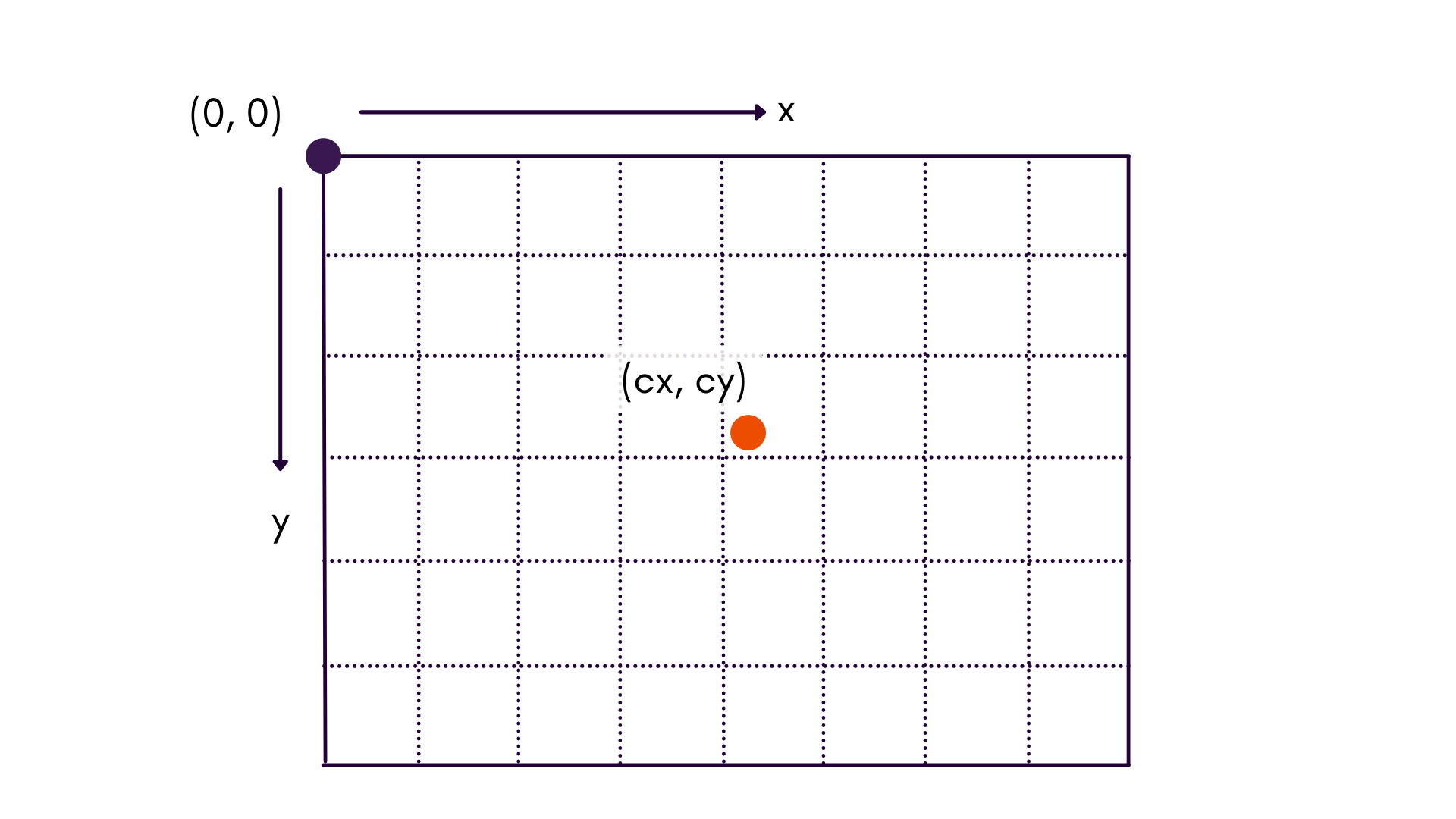This is the standard coordinate system used in the field of computer vision. The origin of the coordinate system is $$(x_0, y_0)$$, and is located in the upper left corner of the image.

When working in this coordinate frame, we use lower-case $$x$$ and $$y$$ to denote that these coordinates are in the image, whereas upper-case $$X$$, $$Y$$, and $$Z$$ are used to denote coordinates of a point in object space.

This coordinate frame is useful when examining feature space, or building histograms across an image.

### UV Coordinate Frame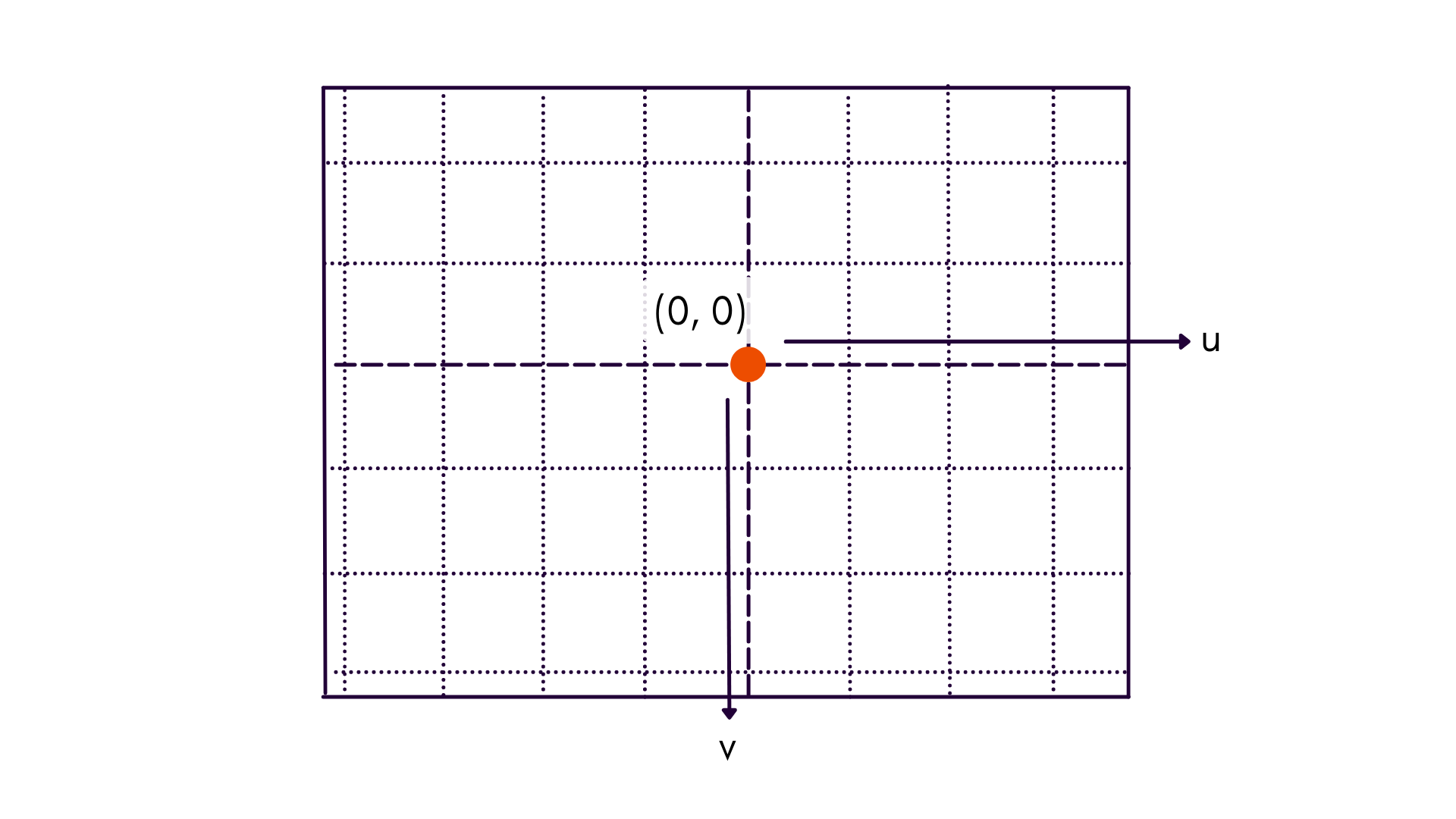This coordinate frame maintains axes conventions, but instead places the origin at the principal point of the image, labeled as $$(u_0, v_0)$$. Notice that the coordinate dimensions are referred to as lower-case $$u$$ and $$v$$, to denote that axes are in image space and relative to the principal point.

Most charts that deal with reprojection error convey more information when plotted in UV coordinates than CV coordinates. For instance, radial distortion increases proportional to radial distance from the principal point... not the top-left of the image.

### Cartesian vs. Polar

In addition to understanding the different origins of our two coordinate frames, we sometimes use polar coordinates in order to be able to visualize reprojections as a function of radial or tangential differences.

When using polar coordinates, we are exclusively dealing with points centered about the principal point. Thus, we go from our previous $$(u, v)$$ frame to $$(r, t)$$.

Cartesian Polar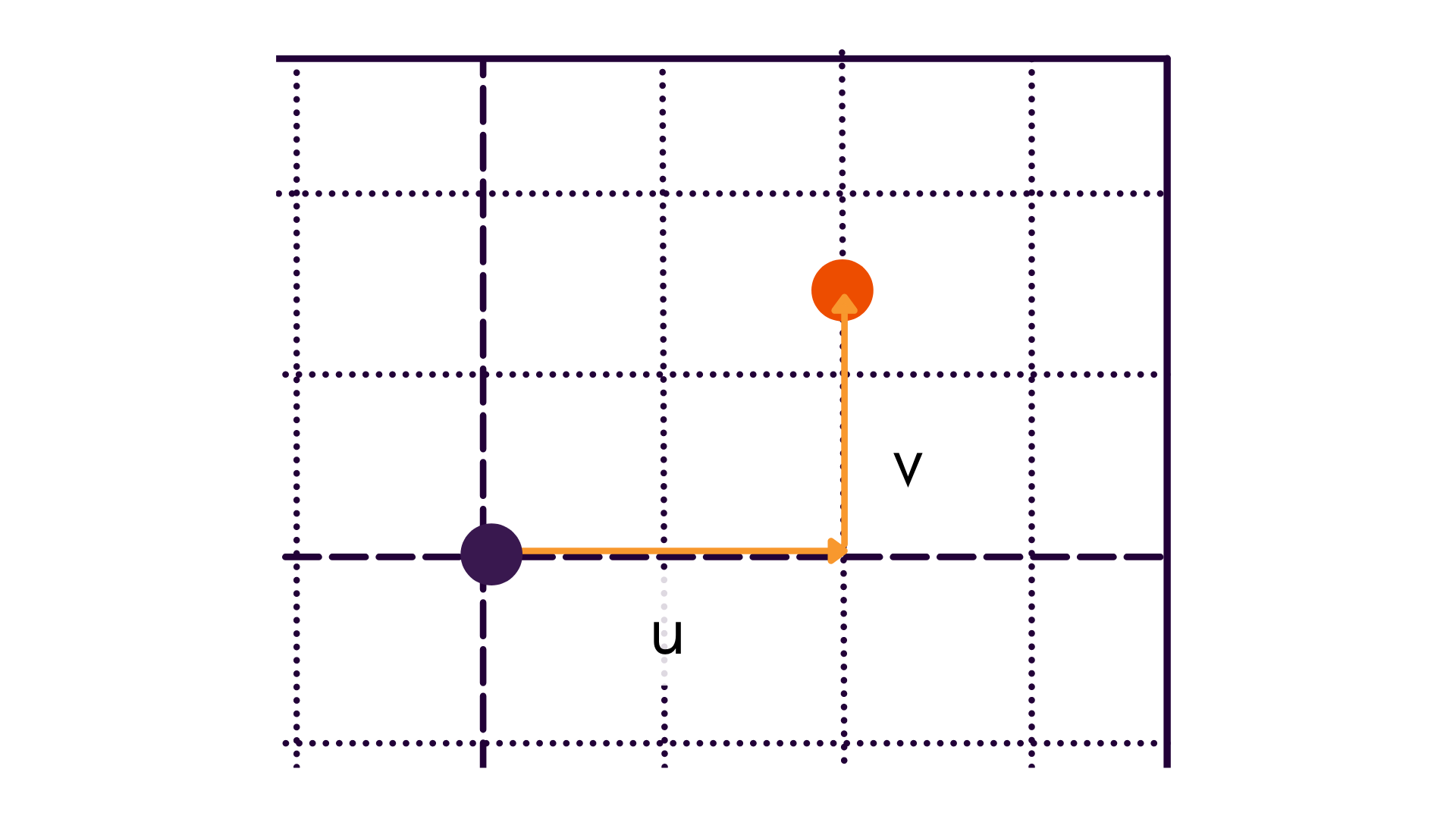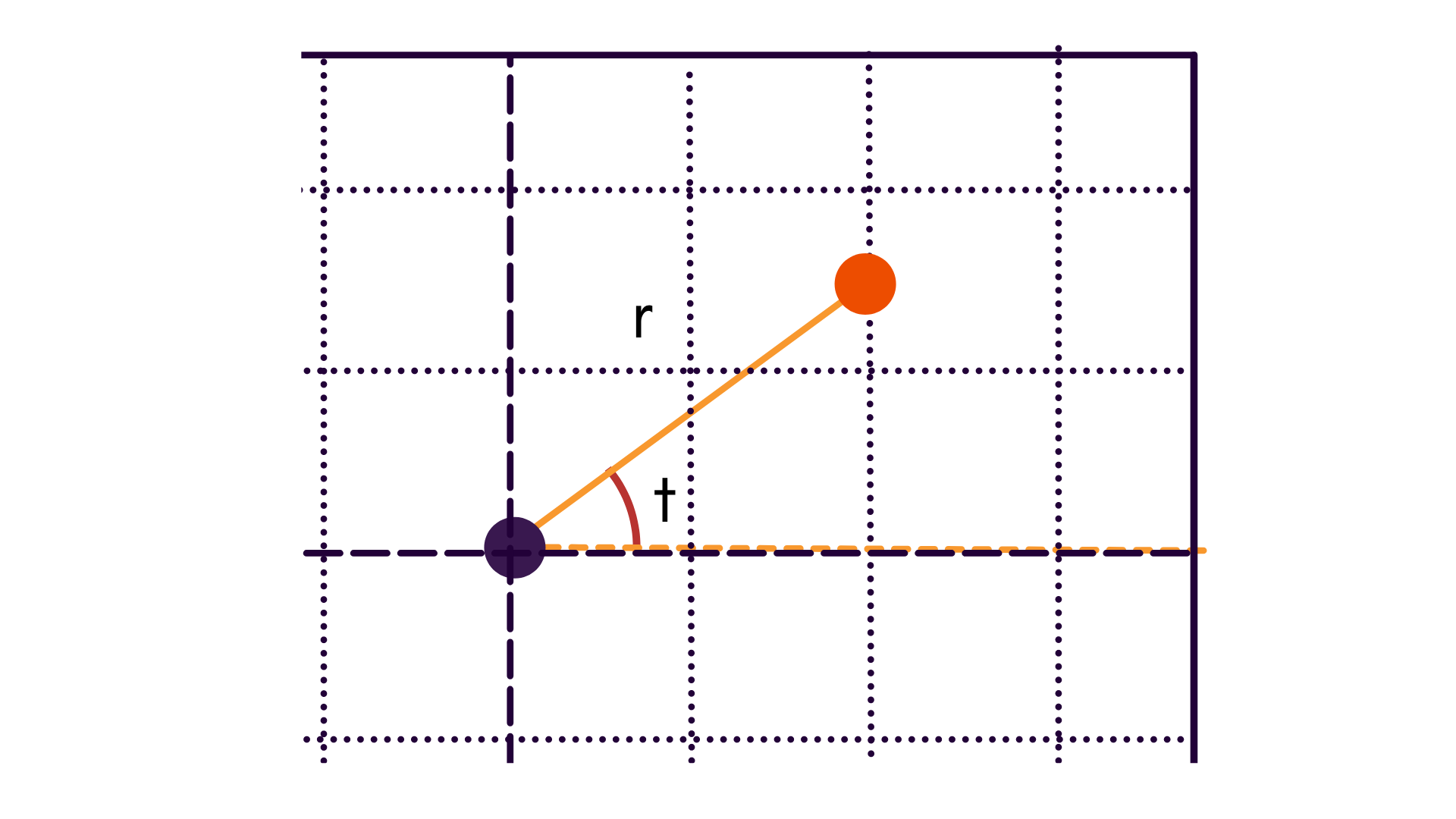## Reprojection Error Analysis

Reprojection errors, more technically referred to as image residuals, are often seen as the only error metric to measure precision within an adjustment. While not the only tool at our disposal, reprojection errors can tell us a lot about the calibration process and provide insight into what image effects were (or were not) properly calibrated for.

Let's dive into some useful reprojection metrics and trends that can be derived from the numbers found in metrics.json.

User Hub Calibration Detail

Every Calibration Detail page in the User Hub provides ready-made visualizations of the metrics discussed here.

### Feature Coverage Analysis

Data Based In: Either CV or UV Coordinate Frame

All image space observations made from a single camera component over the entire calibration process are plotted. This gives us a sense of data coverage over the domain of the image. For a camera calibration process, this chart should ideally have an isometric distribution of points within the image without any large empty spaces. This even spread prevents a camera model from overfitting on any one area.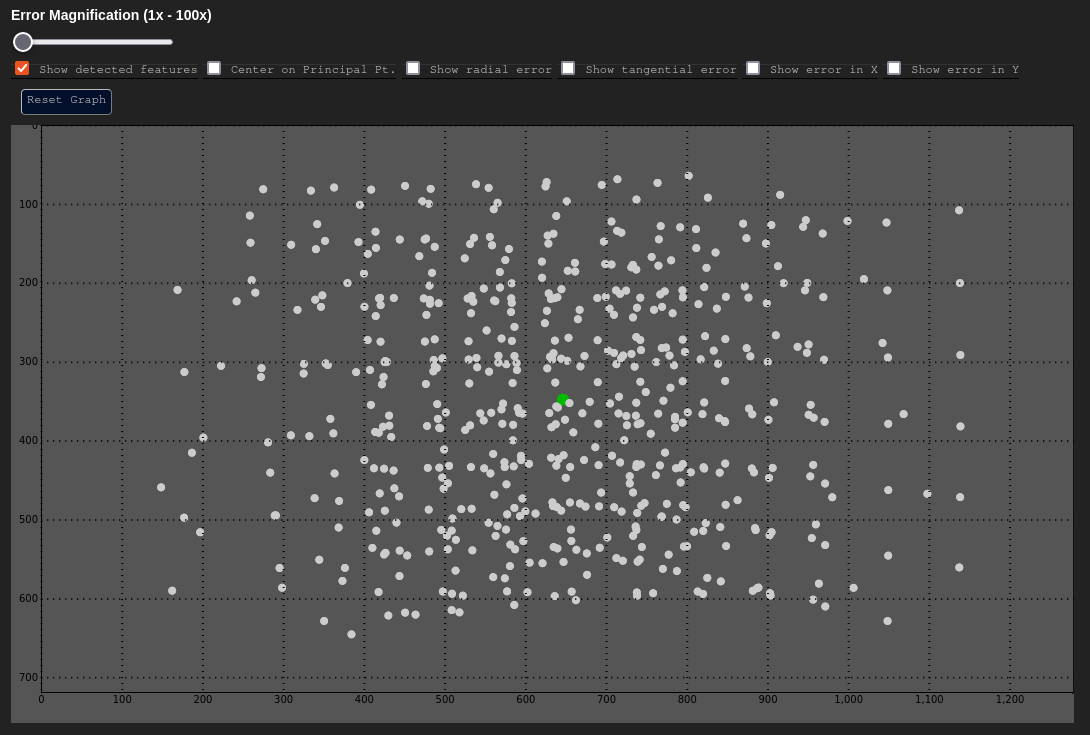In the above example, there are some empty spaces near the periphery of the image. This can happen due to image vignetting (during the capture process), or just merely because one did not move the target to have coverage in that part of the scene.

User Hub Feature Coverage

In addition to just visualizing the coverage, the Calibration Summary in the User Hub also visualizes the reprojection error heatmap of a feature space for radial, tangential, u-wise, and v-wise errors.

### Radial Error - δr vs. r

Data Based In: UV Coordinate Frame

The $$\delta r$$ vs. $$r$$ graph is a graph that plots radial reprojection error as a function of radial distance from the principal point. This graph is an excellent way to characterize distortion error, particularly radial distortions.

Expected Poor Result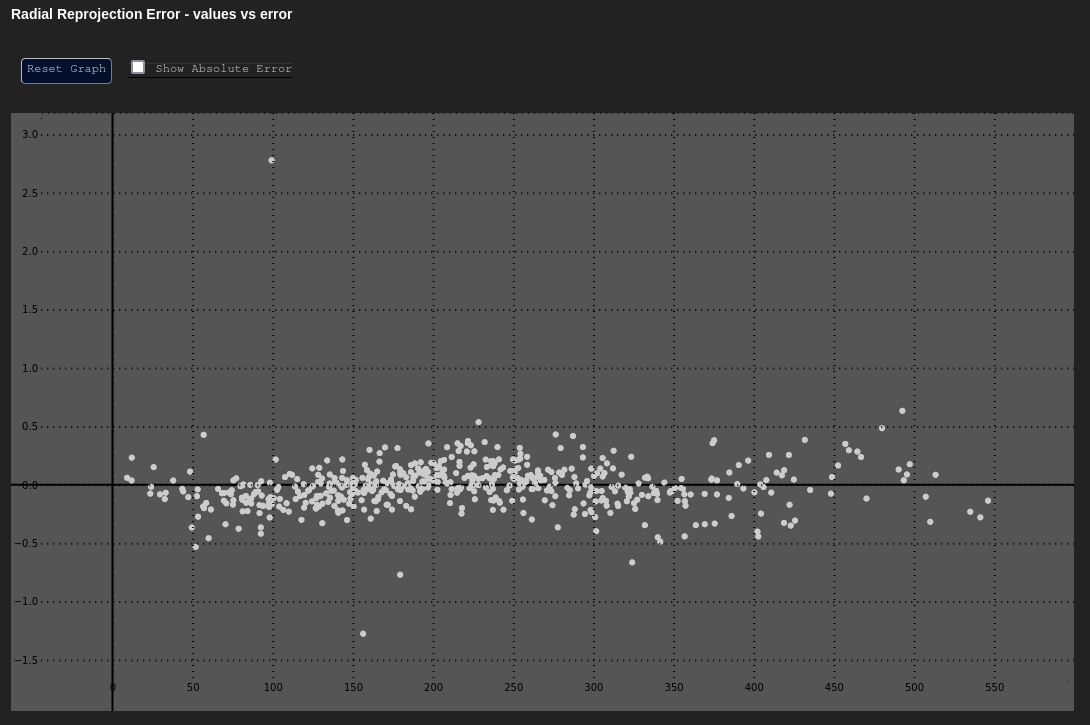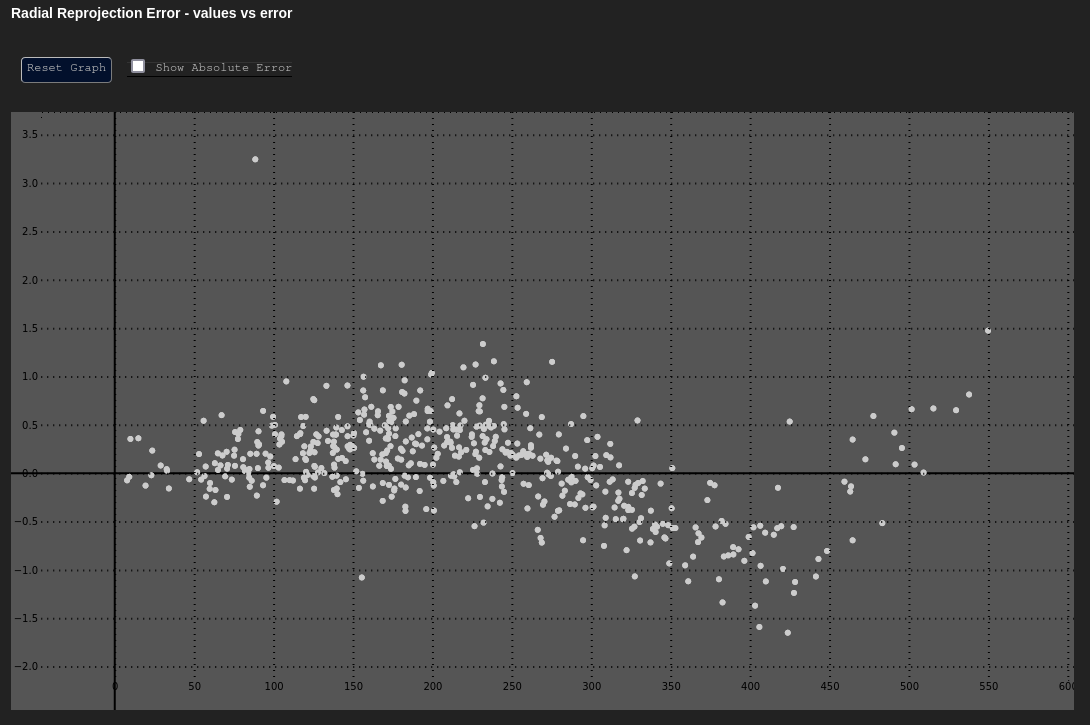Consider the Expected graph above. This distribution represents a fully calibrated system that has modeled distortion using the Brown-Conrady model. The error is fairly evenly distributed and low, even as one moves away from the principal point of the image.

However, if TVCal had been told not to calibrate for a distortion model, the output would look very different (The Poor Result). Radial error fluctuates in a sinusoidal pattern now, getting worse as we move away from the principal point. Clearly, this camera needs some distortion model of some kind in future calibrations.

### Tangential Error - δt vs. t

Data Based In: UV Coordinate Frame

Like the $$\delta r$$ vs. $$r$$ graph, $$\delta t$$ vs. $$t$$ plots the tangential reprojection error as a function of the tangential (angular) component of the data about the principal point.

This can be a useful plot to determine if any unmodeled tangential (de-centering) distortion exists. The Expected chart below shows an adjustment with tangential distortion correctly calibrated and accounted for. The Poor Result shows the same adjustment without tangential distortion modeling applied.

Expected Poor Result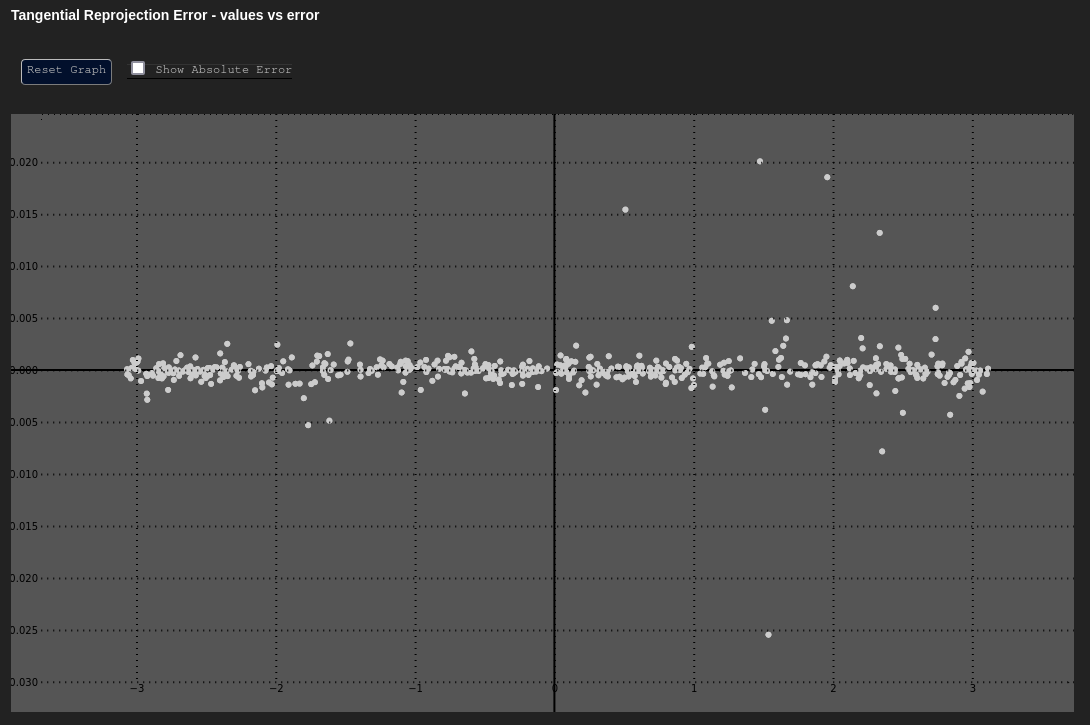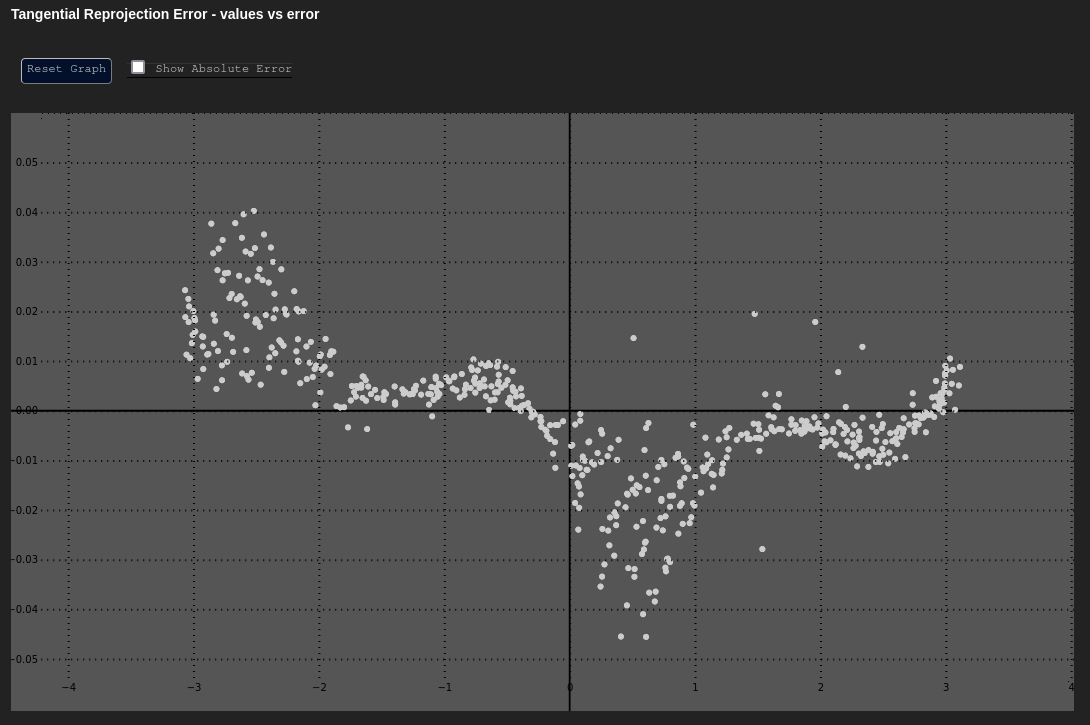### Error in u and v

Data Based In: UV Coordinate Frame

These plot the error in our Cartesian axes ($$\delta u$$ or $$\delta v$$) as a function of the distance along that axis ($$u$$ or $$v$$).

Both of these graphs should have their y-axes centered around zero, and should mostly look uniform in nature. The errors at the extreme edges may be larger or more sparse; however, the errors should not have any noticeable trend.

Expected δu vs. u Expected δv vs. v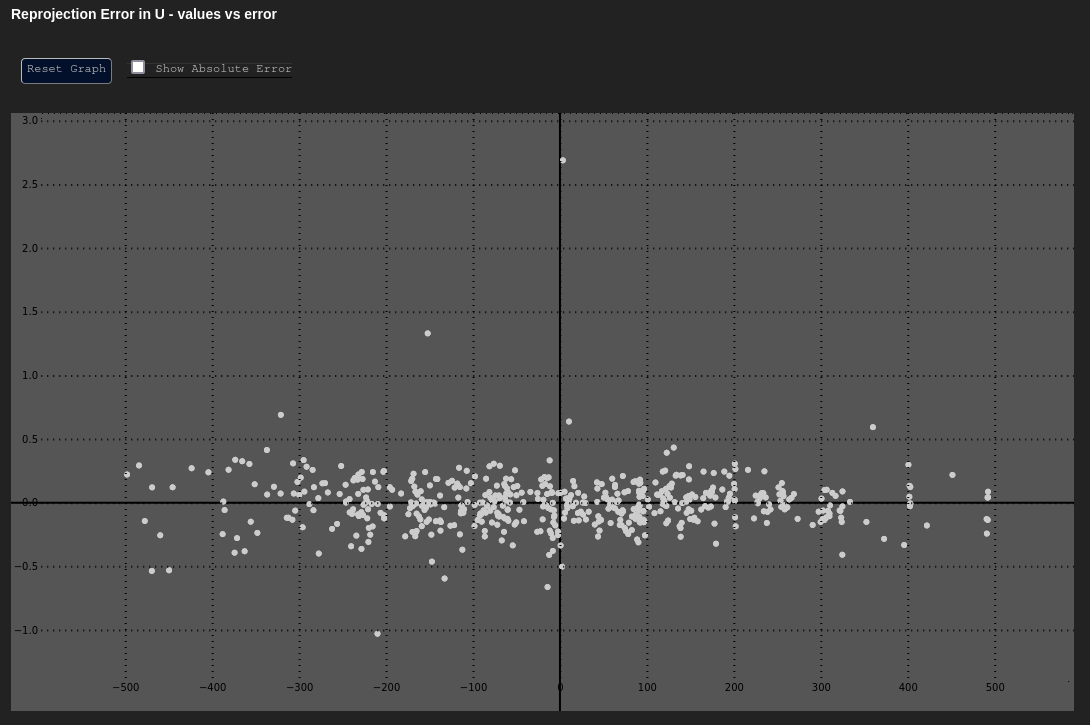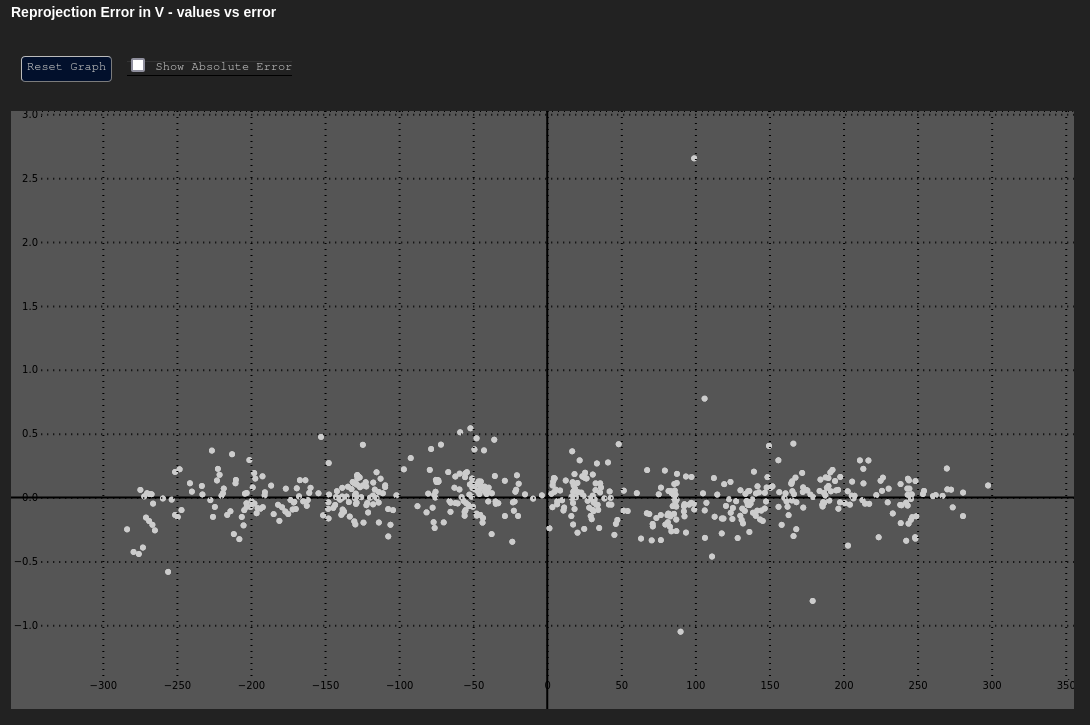## Unmodeled Intrinsics Indicators

There are certain trends and patterns to look out for in the plots above. Many of these can reveal unmodeled intrinsics within a system, like a distortion or affinity that wasn't taken into account in this calibration process. A few of these patterns are outlined below.

### Unmodeled Affinity

A scale affinity distortion that hasn't been calibrated for might look like a linear trend in the data: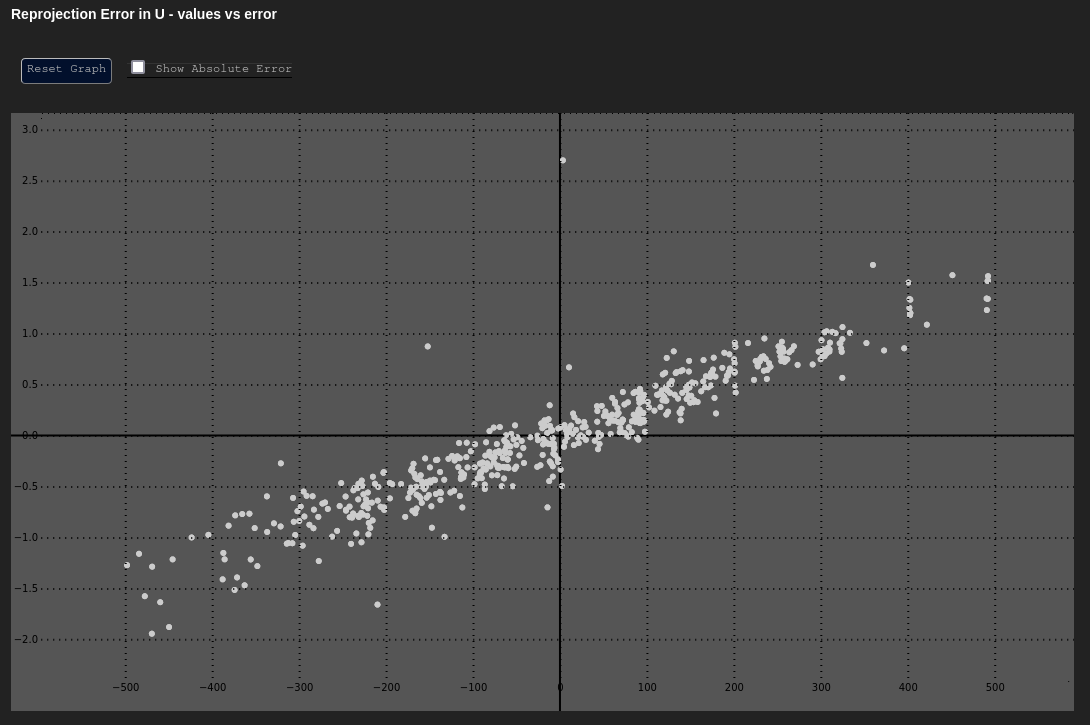However, note that this affine trend may be present in either $$u$$, $$v$$, or both. Alternatively, while the trend above is exaggerated for effect, affinity is often quite small and so may not be visible within the graph itself.

### Unmodeled Tangential Distortion

It was obvious that something was amiss when looking at the Poor Result plot for the $$\delta t$$ vs. $$t$$ graph, above. However, depending on the magnitude of error, we may suspect that any effects we see in such a graph are noise. If we then look at the $$\delta u$$ vs. $$u$$ and $$\delta v$$ vs. $$v$$ graphs, we might see the following trends as well:

Unmodeled Tangential - δu vs. u Unmodeled Tangential - δv vs. v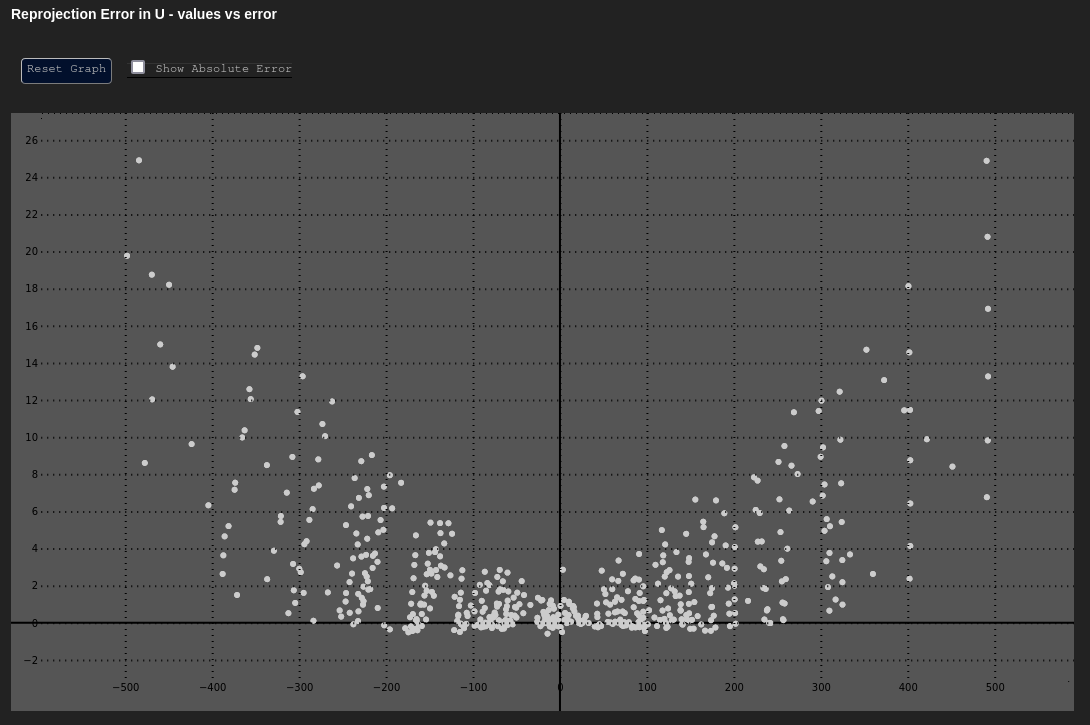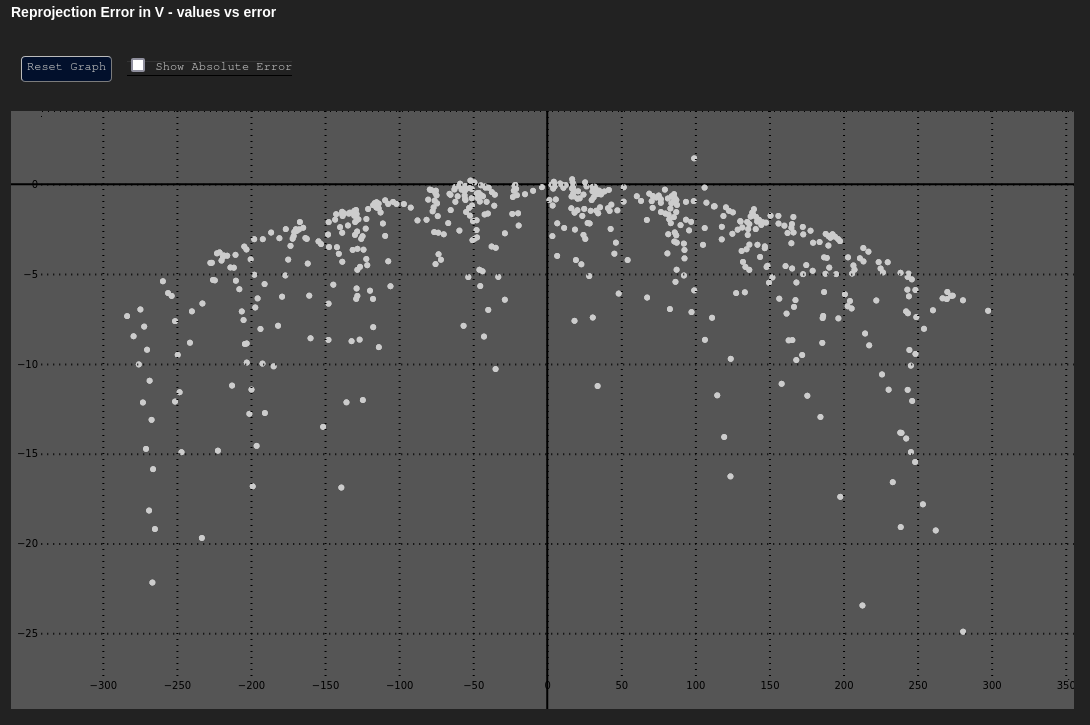## Comparative Analysis

Beyond the above analysis, the charts provided are incredibly useful when comparing calibrations for the same component over time. Comparing these charts temporally is useful when designing a calibration process, and likewise can be useful in deciding between different models (e.g. Brown-Conrady vs. Kannala-Brandt distortion, scale affinity vs. no affinity modeling, etc).

Lastly, comparing these charts across components can be helpful if the components are similar (e.g. similar cameras from the same manufacturer). It is important to keep the axes' scales in mind, however. For example, one cannot compare these charts across two cameras if they have different pixel pitches. A pixel that is 10µm cannot be directly compared to a pixel that is 5µm, as the former is 2 times larger than the latter. One might understandably see that the former component has reprojection errors 2 times smaller than the latter, but this would be a false distinction — that difference is due to the difference in size between the pixels of both cameras.

## Pose Data

Coming Soon

We're still in the process of adding this section. All pose data for every observation can be found in the metrics.json.